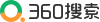# 08-30【Jaap van der Vegt】腾讯会议 特征值问题系列讲座

Department of Applied Mathematics, University of Twente

http://meeting.tencent.com/s/tmQ6pamxaoZi

Eigenvalue problems are important in many applications areas, such as electromagnetics, structural mechanics, quantum theory, and control. Important examples are the Laplace, Maxwell and Schrodinger eigenvalue problems. Finite element methods provide a well-established numerical method to solve eigenvalue problems and are supported by an extensive mathematical theory. In this short course, we will discuss several finite element methods for the computation of eigenvalue problems. Special attention will be given to the theoretical analysis of finite element discretizations for eigenvalue problems. In particular, the convergence theories from Descloux, Nassif and Rapaz, and from Babuska and Osborn will be discussed and used to analyze the convergence of finite element discretizations for eigenvalue problems.